# 最基础的动态数据结构：链表

### 什么是链表？

``class Node {    E e;    Node next;  // 节点中持有下一个节点的引用}``• 优点：真正的动态结构，不需要处理固定容量的问题，从中间插入、删除节点很方便，相较于数组要灵活
• 缺点：丧失了随机访问的能力，不能像数组那种直接通过索引访问

``/** * 链表数据结构实现 **/public class LinkedList<E> {    /**     * 链表中的节点结构     */    private class Node {        E e;        Node next;        public Node() {            this(null, null);        }        public Node(E e) {            this(e, null);        }        public Node(E e, Node next) {            this.e = e;            this.next = next;        }        @Override        public String toString() {            return e.toString();        }    }    /**     * 头节点     */    private Node head;    /**     * 链表中元素的个数     */    private int size;    public LinkedList() {        this.head = null;        this.size = 0;    }    /**     * 获取链表中的元素个数     *     * @return 元素个数     */    public int getSize() {        return size;    }    /**     * 链表是否为空     *     * @return 为空返回true，否则返回false     */    public boolean isEmpty() {        return size == 0;    }    }``

### 在链表中添加元素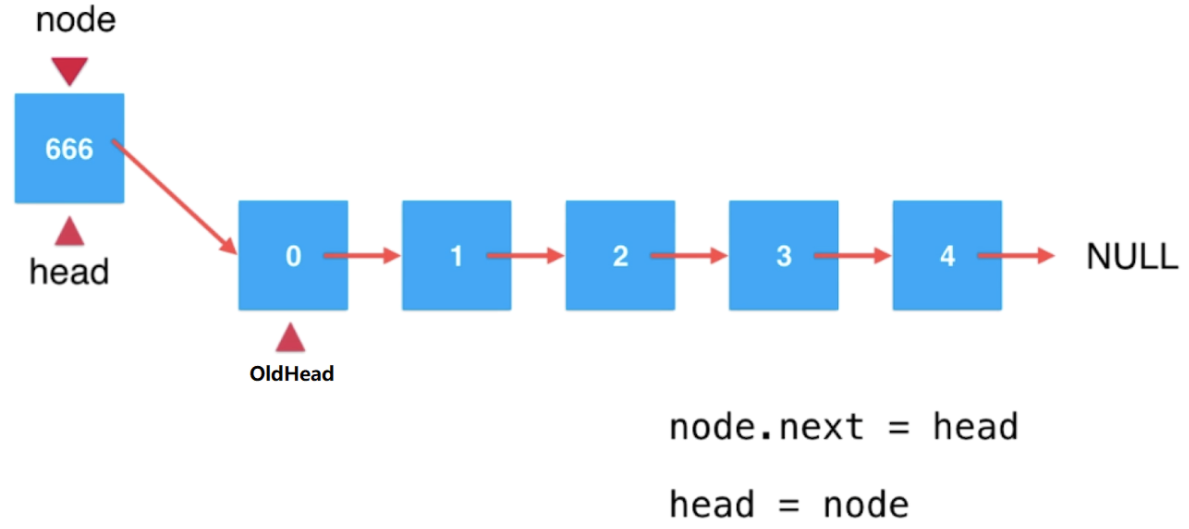``/** * 在链表头添加新的元素e * * @param e 新的元素 */public void addFirst(E e) {    Node node = new Node(e);    node.next = head;    head = node;    // 以上三句代码完全可以直接使用以下一句代码完成，    // 但为了让逻辑更清晰所以这里特地将代码分解了    // head = new Node(e, head);    size++;}``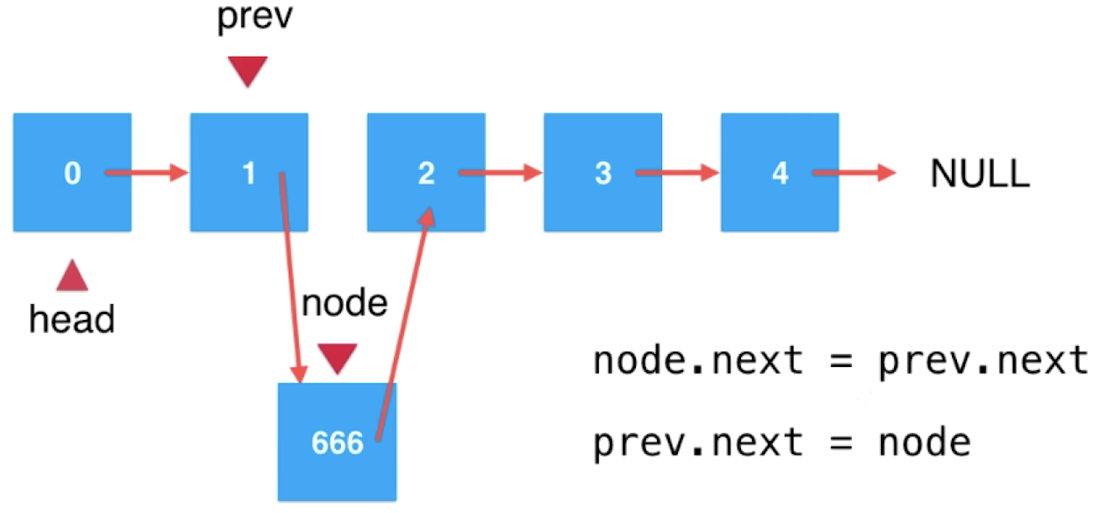``/** * 在链表的index(0-based)位置添加新的元素e * * @param index 元素添加的位置 * @param e     新的元素 */public void add(int index, E e) {    // 检查索引是否合法    if (index < 0 || index > size) {        throw new IllegalArgumentException("Add failed. Illegal index.");    }    // 链表头添加需特殊处理    if (index == 0) {        addFirst(e);    } else {        Node prev = head;        // 移动prev到index - 1的位置        for (int i = 0; i < index - 1; i++) {            prev = prev.next;        }        Node node = new Node(e);        node.next = prev.next;        prev.next = node;        // 同样，以上三句代码可以一句代码完成        // prev.next = new Node(e, prev.next);        size++;    }}``

``/** * 在链表末尾添加新的元素e * * @param e 新的元素 */public void addLast(E e) {    add(size, e);}``

### 使用链表的虚拟头节点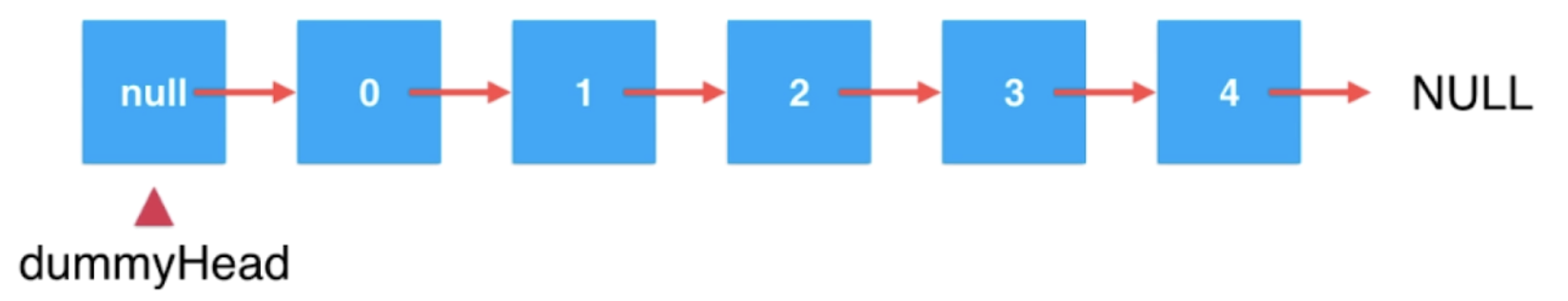``public class LinkedList<E> {    /**     * 虚拟头节点     */    private Node dummyHead;    /**     * 链表中元素的个数     */    private int size;    public LinkedList() {        this.dummyHead = new Node(null, null);        this.size = 0;    }    /**     * 在链表的index(0-based)位置添加新的元素e     *     * @param index 元素添加的位置     * @param e     新的元素     */    public void add(int index, E e) {        if (index < 0 || index > size) {            throw new IllegalArgumentException("Add failed. Illegal index.");        }        Node prev = dummyHead;        // 移动prev到index前一个节点的位置        for (int i = 0; i < index; i++) {            prev = prev.next;        }        Node node = new Node(e);        node.next = prev.next;        prev.next = node;        // 同样，以上三句代码可以一句代码完成        // prev.next = new Node(e, prev.next);        size++;    }    /**     * 在链表头添加新的元素e     *     * @param e 新的元素     */    public void addFirst(E e) {        add(0, e);    }    /**     * 在链表末尾添加新的元素e     *     * @param e 新的元素     */    public void addLast(E e) {        add(size, e);    }}``

### 链表的遍历、查询和修改

``/** * 获取链表的第index(0-based)个位置的元素 * * @param index * @return */public E get(int index) {    if (index < 0 || index >= size) {        throw new IllegalArgumentException("Get failed. Illegal index.");    }    Node cur = dummyHead.next;    for (int i = 0; i < index; i++) {        cur = cur.next;    }    return cur.e;}/** * 获取链表中的第一个元素 * * @return */public E getFirst() {    return get(0);}/** * 获取链表中的最后一个元素 * * @return */public E getLast() {    return get(size - 1);}/** * 修改链表的第index(0-based)个位置的元素为e * * @param index * @param e */public void set(int index, E e) {    if (index < 0 || index >= size) {        throw new IllegalArgumentException("Set failed. Illegal index.");    }    Node cur = dummyHead.next;    for (int i = 0; i < index; i++) {        cur = cur.next;    }    cur.e = e;}/** * 查找链表中是否包含元素e * * @param e * @return */public boolean contain(E e) {    Node cur = dummyHead.next;    // 第一种遍历链表的方式    while (cur != null) {        if (cur.e.equals(e)) {            return true;        }        cur = cur.next;    }    return false;}@Overridepublic String toString() {    if (isEmpty()) {        return "[]";    }    StringBuilder sb = new StringBuilder();    sb.append(String.format("LinkedList: size = %d\n", size));    sb.append("[");    Node cur = dummyHead.next;    // 第二种遍历链表的方式    for (int i = 0; i < size; i++) {        sb.append(cur.e).append(" -> ");        cur = cur.next;    }    // 第三种遍历链表的方式    // for (Node cur = dummyHead.next; cur != null; cur = cur.next) {    //     sb.append(cur.e).append(" -> ");    // }    return sb.append("NULL]").toString();}``

### 从链表中删除元素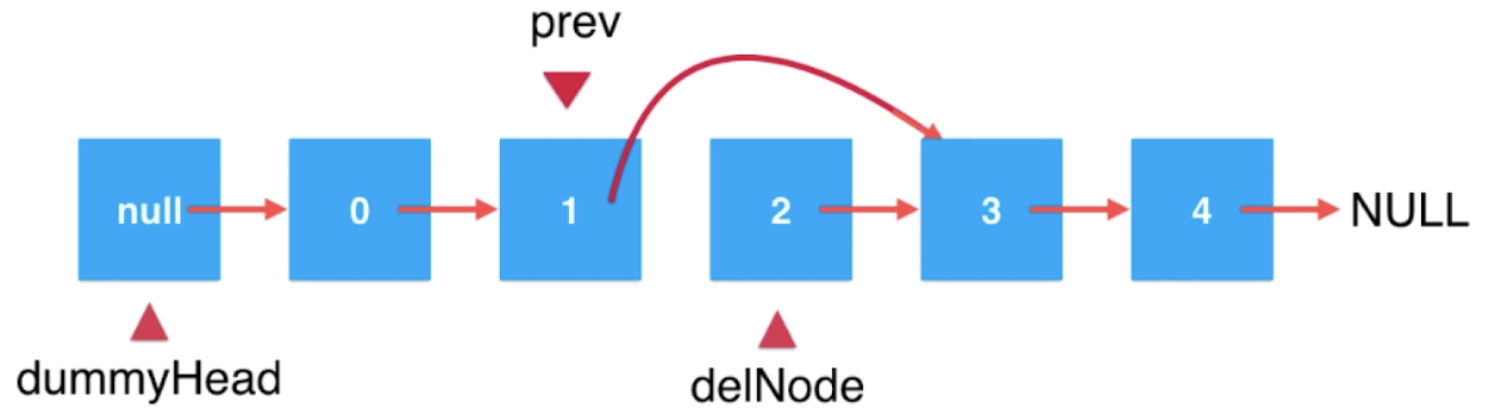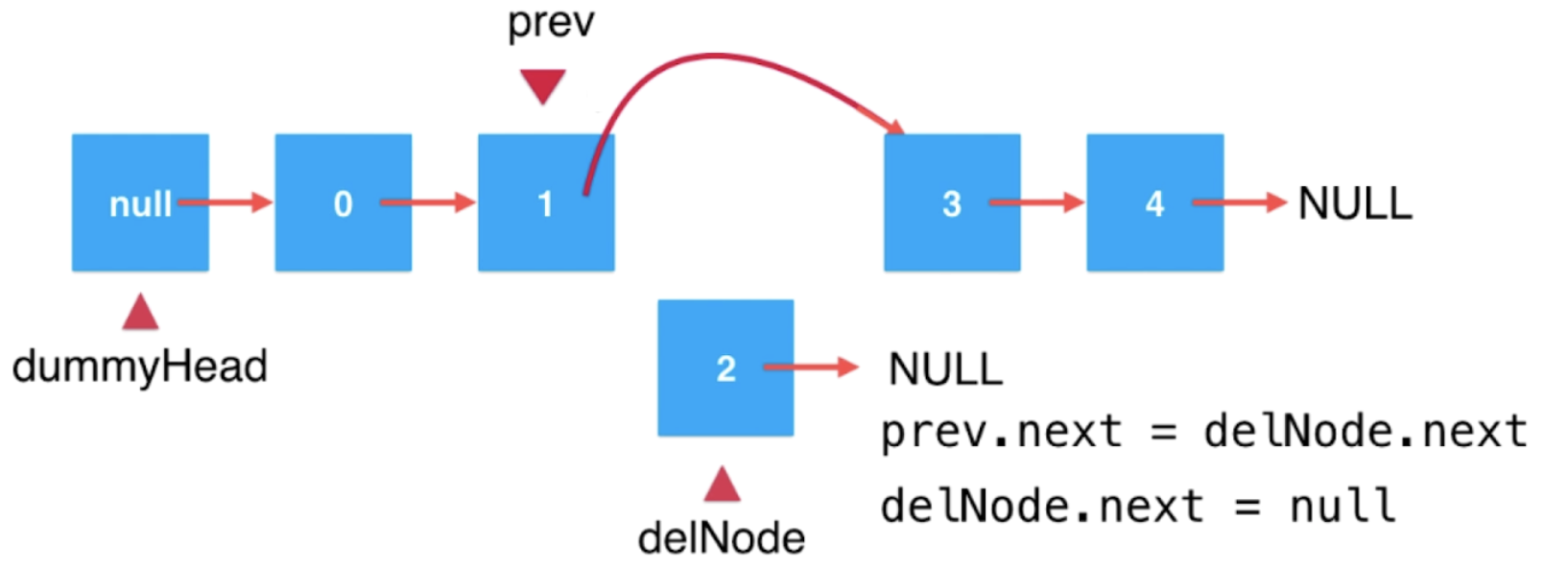``/** * 从链表中删除第index(0-based)个位置的元素，并返回删除的元素 * * @param index * @return 被删除的节点所存储的元素 */public E remove(int index) {    if (index < 0 || index >= size) {        throw new IllegalArgumentException("remove failed. Illegal index.");    }    Node prev = dummyHead;    for (int i = 0; i < index; i++) {        prev = prev.next;    }    Node delNode = prev.next;    // 把引用改变一下就完成了删除    prev.next = delNode.next;    delNode.next = null;    size--;    return delNode.e;}``

``/** * 删除链表中第一个元素 * * @return 被删除的元素 */public E removeFirst() {    return remove(0);}/** * 删除链表中最后一个元素 * * @return 被删除的元素 */public E removeLast() {    return remove(size - 1);}``

``````addLast(e)         // O(n)
removeLast()       // O(n)
removeFirst()      // O(1)
remove(index)      // O(n)
set(index, e)      // O(n)
get(index)         // O(n)
contain(e)         // O(n)
``````

### 使用链表实现栈

``/** * 基于链表实现栈数据结构 **/public class LinkedListStack<E> implements Stack<E> {    private LinkedList<E> linkedList;    public LinkedListStack() {        this.linkedList = new LinkedList<>();    }    @Override    public int getSize() {        return linkedList.getSize();    }    @Override    public boolean isEmpty() {        return linkedList.isEmpty();    }    @Override    public void push(E e) {        linkedList.addFirst(e);    }    @Override    public E pop() {        return linkedList.removeFirst();    }    @Override    public E peek() {        return linkedList.getFirst();    }    @Override    public String toString() {        if (isEmpty()) {            return "[]";        }        StringBuilder sb = new StringBuilder();        sb.append(String.format("LinkedListStack: size = %d\n", getSize()));        sb.append("top [");        for (int i = 0; i < getSize(); i++) {            sb.append(linkedList.get(i));            if (i != getSize() - 1) {                sb.append(", ");            }        }        return sb.append("]").toString();    }    // 测试    public static void main(String[] args) {        Stack<Integer> stack = new LinkedListStack<>();        for (int i = 0; i < 5; i++) {            stack.push(i);            System.out.println(stack);        }        stack.pop();        System.out.println(stack);    }}``

### 带有尾指针的链表：使用链表实现队列

``/** * 基于链表实现的队列数据结构 **/public class LinkedListQueue<E> implements Queue<E> {    private class Node {        E e;        Node next;        public Node() {            this(null, null);        }        public Node(E e) {            this(e, null);        }        public Node(E e, Node next) {            this.e = e;            this.next = next;        }        @Override        public String toString() {            return e.toString();        }    }    /**     * 头节点     */    private Node head;    /**     * 尾节点     */    private Node tail;    /**     * 表示队列中的元素个数     */    private int size;    @Override    public void enqueue(E e) {        if (tail == null) {            // 链表没有元素            tail = new Node(e);            head = tail;        } else {            // 链尾入队元素            tail.next = new Node(e);            tail = tail.next;        }        size++;    }    @Override    public E dequeue() {        if (isEmpty()) {            throw new IllegalArgumentException("Can't dequeue from an empty queue.");        }        // 链首出队元素        Node retNode = head;        head = head.next;        retNode.next = null;        if (head == null) {            // 队列里没元素的话，尾节点需要置空            tail = null;        }        size--;        return retNode.e;    }    @Override    public E getFront() {        if (isEmpty()) {            throw new IllegalArgumentException("Queue is empty.");        }        return head.e;    }    @Override    public int getSize() {        return size;    }    @Override    public boolean isEmpty() {        return size == 0;    }    @Override    public String toString() {        if (isEmpty()) {            return "[]";        }        StringBuilder sb = new StringBuilder();        sb.append(String.format("LinkedListQueue: size = %d\n", getSize()));        sb.append("front [");        Node cur = head;        while (cur != null) {            sb.append(cur.e).append(", ");            cur = cur.next;        }        return sb.append("NULL] tail").toString();    }}``

##### 热门推荐
•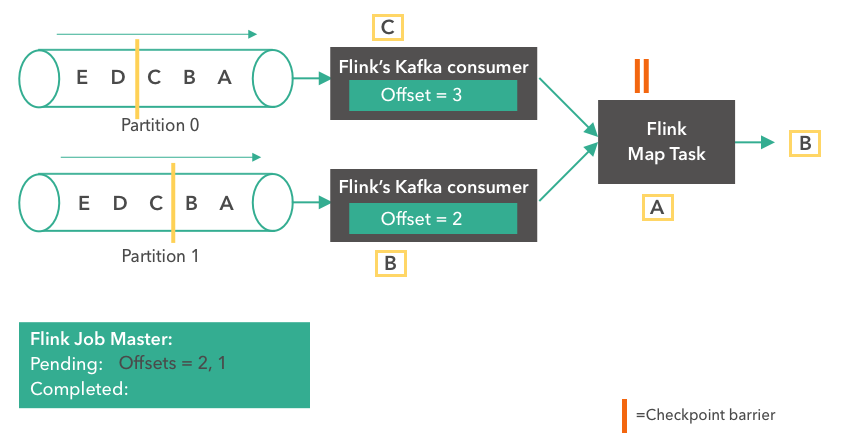浏览(2,357)
•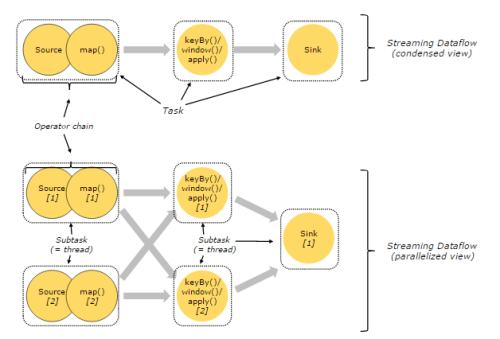浏览(1,659)
•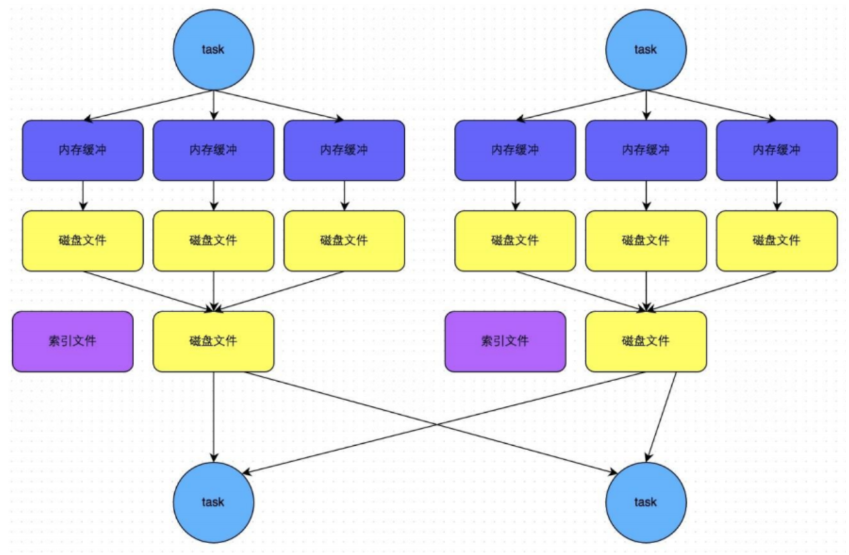浏览(1,646)
•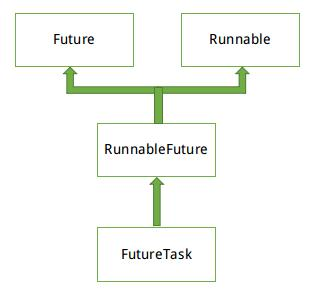浏览(1,568)
•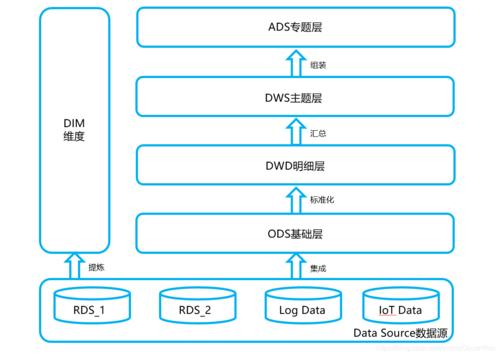浏览(1,562)
•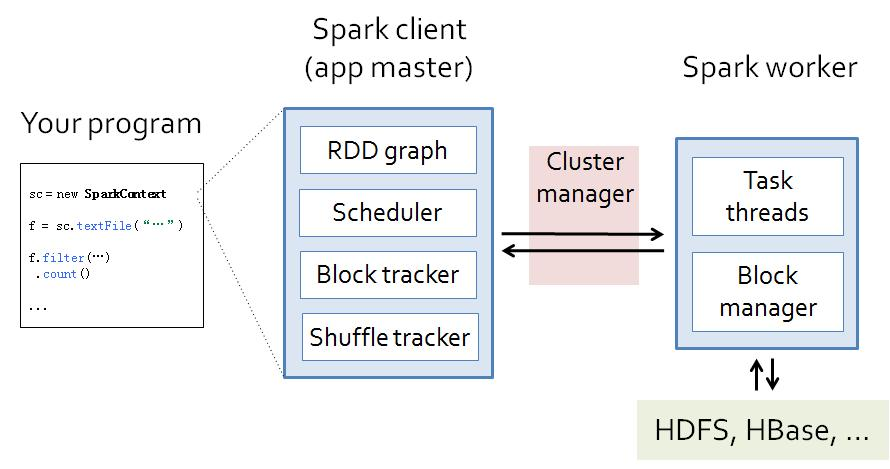浏览(1,539)
•浏览(1,499)
•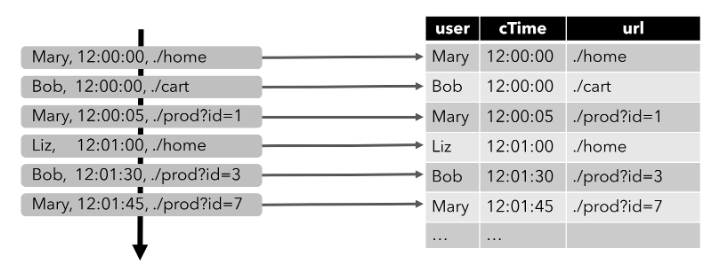浏览(1,437)
•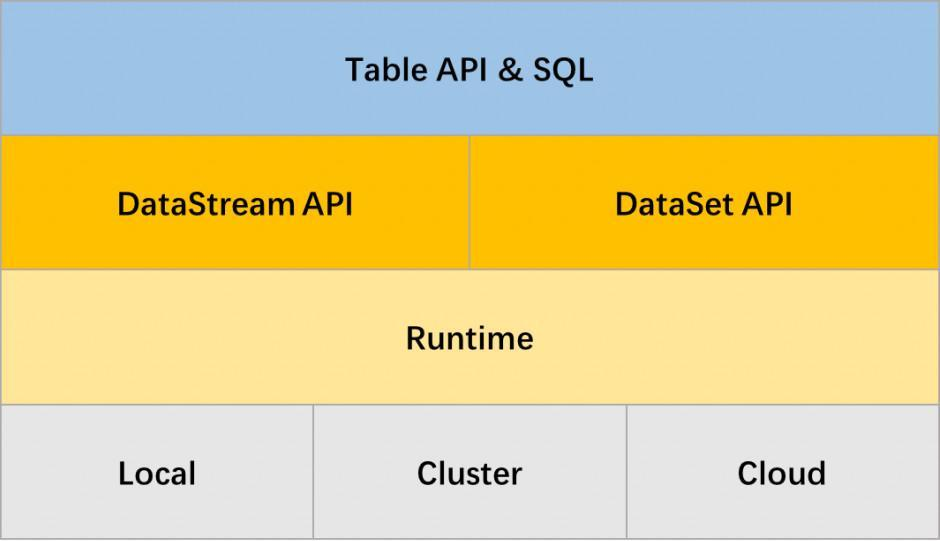浏览(1,429)
•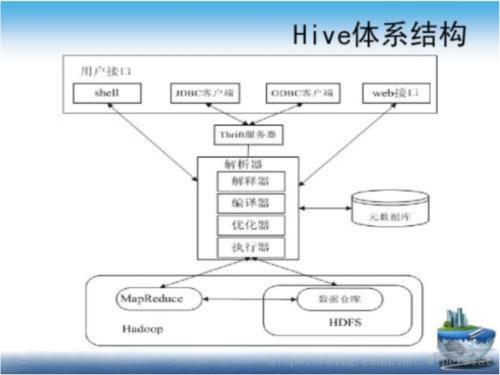浏览(1,399)